Select Page

Looking to understand What the e in Math is for? Look No further!

Suppose you put $1 in a bank. The bank pays 4% interest a year, and this is credited to your account at the end of a year. A little thought shows that at the end of five years an amount of money equal to$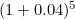will sit in the bank (this bank charges no fees).

However, if the interest (still at an annual rate of 4%) was “compounded” every quarter, then the amount at the end of five years would be $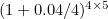. If the bank gave an interest rate of 100% annually, then after one year the bank balance would then be$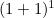, and if the interest were compounded quarterly it would be $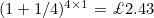. If you were even luckier and found a bank that compounded monthly, the 100% annual rate of interest would then gives you$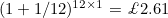after one year. Likewise, daily compounding would give you \$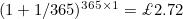.

It’s obvious that compounding more frequently results in more money in the bank. So it is natural to ask whether compounding at every instant in time (that is, continuously) leads to an infinite amount in the bank.

To answer this question we need to evaluate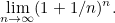This quantity turns out again to be– the same base value with the property that the gradient of the graph is unity at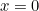.

Now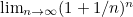can be expanded very nicely using the trusty old Binomial Theorem. We find that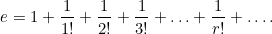This series is convergent, and evaluating the sum far enough to give no change in the fourth decimal place (this occurs after the seventh term is added) gives an approximation forof 2.718.

It was that great mathematician Leonhard Euler who discovered the number e and calculated its value to 23 decimal places. It is often called Euler’s number and, like pi, is a transcendental number (this means it is not the root of any algebraic equation with integer coefficients). Its properties have led to it as a “natural” choice as a logarithmic base, and indeed e is also known as the natural base or Naperian base (after John Napier).

There is the remarkable property that if the function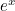(known as the exponential function and also denoted as “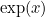“) is differentiated with respect to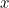, then the result is the same function. The proof of this can be seen in many textbooks on elementary calculus. It’s also true that for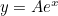,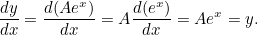In fact, it is true that given the equation: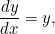then a solution for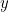iswhere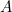is a constant.

It’s also possible to relate the general exponential function,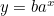, to the exponential function. This is because some numbercan always be chosen so that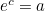. Then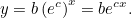Differentiating with respect togives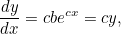which tells us that the general exponential relation,, that so often crops up in applications, is in fact a solution of the differential equation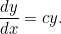(Actually it happens to be the only type of solution to this important equation.)

It is this equation that emerges naturally when attempting to model various processes.

If you have any other Math related questions, drop us a comment and we will endeavor to get back to you or check if we already have answered it for you.

Parse error: syntax error, unexpected '.', expecting '&' or variable (T_VARIABLE) in /home/intellecquity/public_html/wp-content/plugins/ad-inserter/includes/agent/Agent.php on line 357